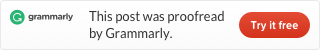# How to categorize and group data in time intervals with Power QueryIf you have DateTime or time column in your data, then sometimes there is a desire to analyze it by time intervals. With Excel and DAX functions you have plenty of possibilities how to do that, but in Power Query, you have to sweat a little bit. Here is the way how to do that in Power Query.In Power Query time is stored like decimal number between 0 and 1.
First of all, you need to calculate how many necessary time intervals you have in 24 hour period, then multiply with a decimal number that represents time, round to nearest time interval and convert back to time by dividing with time intervals.

With all this in mind rounding to the nearest 30-minute interval goes like this.
`=Time.From(Number.RoundDown(Number.From(Time.From([DateTime]))*(24*60/30))/(24*60/30))`

Or for the rounding to the nearest 15-minute interval, it goes like this.
`=Time.From(Number.RoundDown(Number.From(Time.From([DateTime]))*(24*60/15))/(24*60/15))`

### 1 comment on “How to categorize and group data in time intervals with Power Query”

1.M

Exactly what I needed, exactly when I needed it!